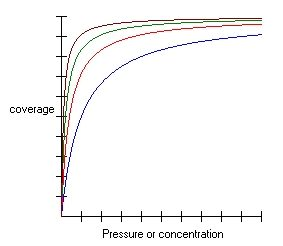# Langmuir Isotherm Calculator

Created by Łucja Zaborowska, MD, PhD candidate
Reviewed by Bogna Szyk and Jack Bowater
Last updated: Jun 05, 2023

The Langmuir isotherm calculator is an easy-to-use tool for computing the fraction or percent of the adsorbent surface covered by an adsorbate using the Langmuir model.

Our Langmuir Isotherm calculator allows you to use either the partial pressure or the molarity of the substance, depending whether you're calculating the isotherm for a gas or for a liquid.

## What is Langmuir adsorption isotherm?

Firstly, we'd like to remind you of a few basic definitions:

• Adsorption – is a process of adhesion that connects molecules, atoms, or ions to a surface (or a phase boundary).

• In this process, the adsorbate creates a thin layer on the surface of the adsorbent.

• Chemisorption – a type of adsorption that creates strong chemical bonds between the adsorbate and the adsorbent.

• Isotherm – is a function that describes the change in the amount of adhered substance, depending on the substance's partial pressure or concentration. The reaction must take place in isothermal conditions (that is, with constant temperature). 🌡️ If needed, you can use the temperature conversion tool as well.

Coming back to the Langmuir theory:

The Langmuir isotherm assumes that the adsorbate can only create a monolayer of particles on the adsorbent's surface. These particles interact only with the adsorbent (vertical interactions) and do not interact with each other (horizontal interactions).

Langmuir isotherm is based on these assumptions:

• The adsorbate particles behave as an ideal gas;
• The energy of the surface is homogeneous (identical and flat in all the places); and
• The adsorbate particles can not create multi-layers.

Read more on the characteristics of this adhesion process in the section below 📄

🙋 Want to know how ideal gas behaves? Check out our ideal gas law calculator.

## How to calculate Langmuir isotherm?

We understand that even the best Langmuir isotherm calculators can not replace actual knowledge. In this section, you can discover the formula we use in every detail.

Our tool uses the following Langmuir isotherm equation:

$\theta = \frac{\text K_\text{eq} \times \text P}{1+(\text K_\text {eq} \times \text P)}$

where:

• $\theta$ – Draction of the surface covered by adsorbate;
• $\text K_\text{eq}$ – Equilibrium constant of adsorption; and
• $\text P$ – Partial pressure of the gas or the molar concentration of the liquid.

In order to receive the percentage of the surface covered by the adsorbate, use the equation below:

$\theta_\text{percent}= \theta_\text{fraction} \times 100$

You might want to take a look at our equilibrium constant calculator if you would like a better understanding of the topic.

## How to plot the Langmuir adsorption isotherm?

This is one of the most common questions regarding the adsorption isotherm; in order to answer it, we first need to understand the rules of the Langmuir adsorption model.

We have a partial pressure calculator that helps you determine and understand partial pressure.

• The adsorbate particles hit the surface of the adsorbent, just like little bullets hitting the target. The greater the surface, the bigger the target, and the easier it is to hit the target.

• Imagine that we're increasing the concentration (or partial pressure) of the mixture - this results in us having more bullets to shoot, so the probability of hitting the target (and adhesion!) increases.

• After a while, however, the shooting target becomes full of bullets and cannot accept any new shots. That's exactly what's happening to the adsorbent surface - it becomes filled with particles since it can form only one layer.

As a result, the Langmuir isotherm graph is pretty steep at the beginning when we increase the pressure or concentration, supplying the system with new bullets. After a while, however, all the places on the surface of the adsorbent are taken. The coverage becomes full – as a result, the graph becomes flat.Łucja Zaborowska, MD, PhD candidate
Equilibrium constant (K eq)
Partial pressure (P)
Pa
Surface fraction (θ)
Surface percent
%
People also viewed…

### Calibration curve

With our calibration curve calculator, you will be able to find out the concentration of an unknown sample in a few seconds!

### Christmas tree

Welcome to the Christmas tree calculator, where you will find out how to decorate your Christmas tree in the best way. Take a look at the perfect Christmas tree formula prepared by math professors and improved by physicists. Plan in advance how many lights and decorations you'll need!

### Meat footprint

Check out the impact meat has on the environment and your health.

### Water hardness

Estimate the water hardness based on the calcium and magnesium concentration using the water hardness calculator.